# = surviving chem 2!! Ah really O ACIDS AND BASES Calculating the pH of a weak...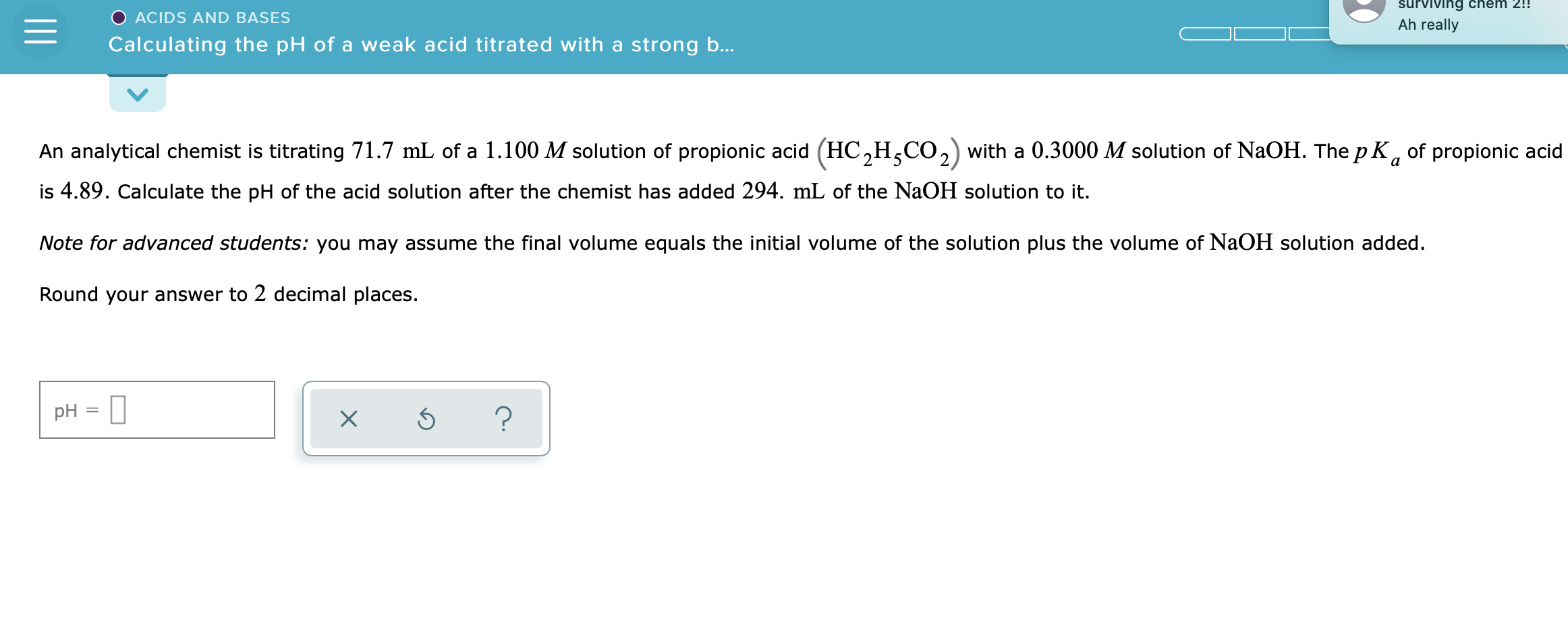= surviving chem 2!! Ah really O ACIDS AND BASES Calculating the pH of a weak acid titrated with a strong b... An analytical chemist is titrating 71.7 mL of a 1.100 M solution of propionic acid (HC,H,CO2) with a 0.3000 M solution of NaOH. The pK, of propionic acid is 4.89. Calculate the pH of the acid solution after the chemist has added 294. mL of the NaOH solution to it. Note for advanced students: you may assume the final volume equals the initial volume of the solution plus the volume of NaOH solution added. Round your answer to 2 decimal places. pH = 1 | xs ?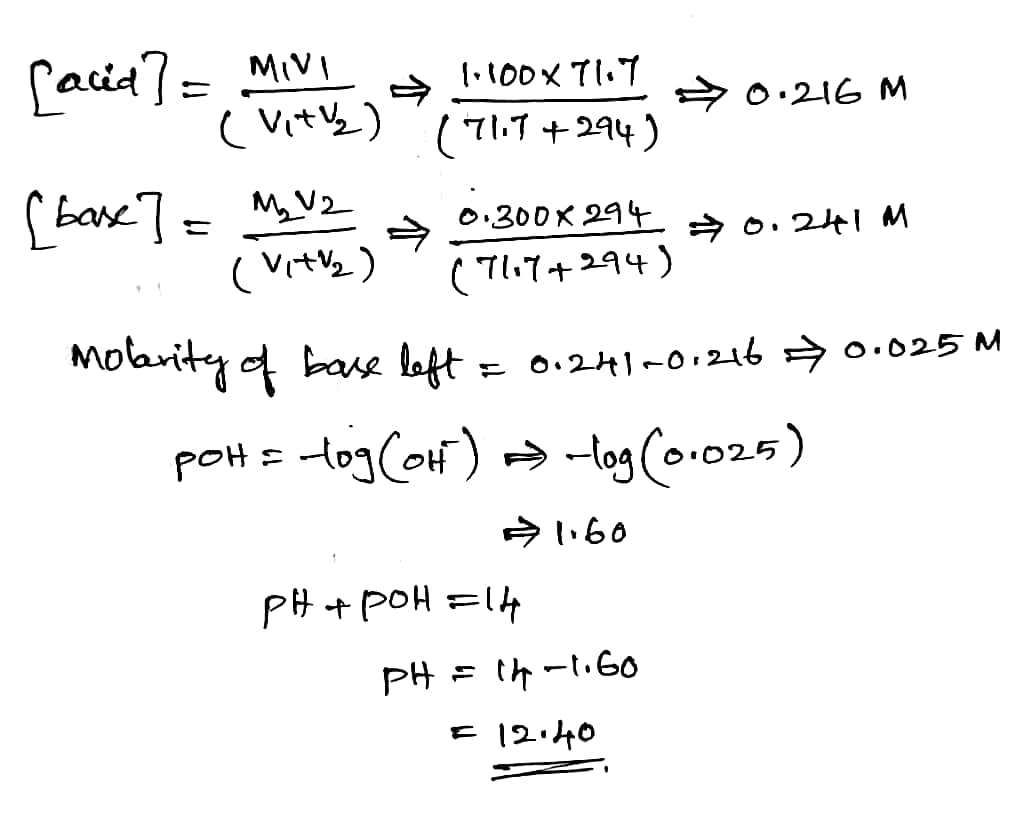#### Earn Coin

Coins can be redeemed for fabulous gifts.

Similar Homework Help Questions
• ### O ACIDS AND BASES Calculating the pH of a weak acid titrated with a strong base...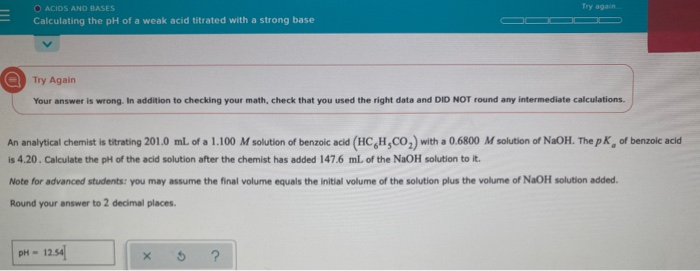O ACIDS AND BASES Calculating the pH of a weak acid titrated with a strong base Try Again Your answer is wrong. In addition to checking your math, check that you used the right data and DID NOT round any intermediate calculations. An analytical chemist is titrating 2010 ml of a 1.100 M solution of benzoic acid (HCH,CO) with a 0.6800 M solution of NaOH. The pK, of benzoic acid is 4.20. Calculate the pH of the acid solution after...

• ### O ACIDS AND BASES Calculating the pH of a weak acid titrated with a strong base...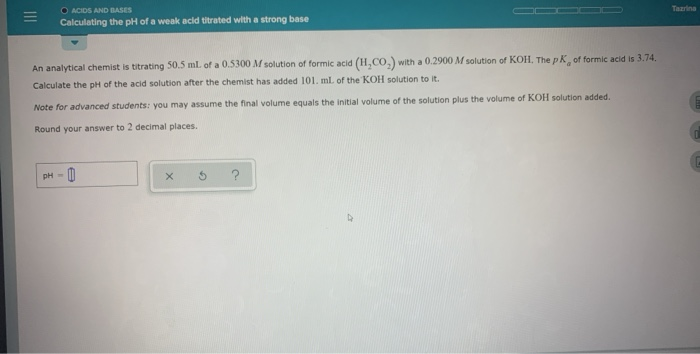O ACIDS AND BASES Calculating the pH of a weak acid titrated with a strong base An analytical Chemist is titrating 50.5 ml of a 0.5300 M solution of formic acid (H.CO.) with a 0.2900 M solution of KOH. The pk of formic acid is 3.74 Calculate the pH of the acid solution after the chemist has added 101. ml of the KOH solution to it. Note for advanced students you may assume the final volume equals the initial volume...

• ### calculating the ph of a weak acid titrated with a strong base An analytical chemist is...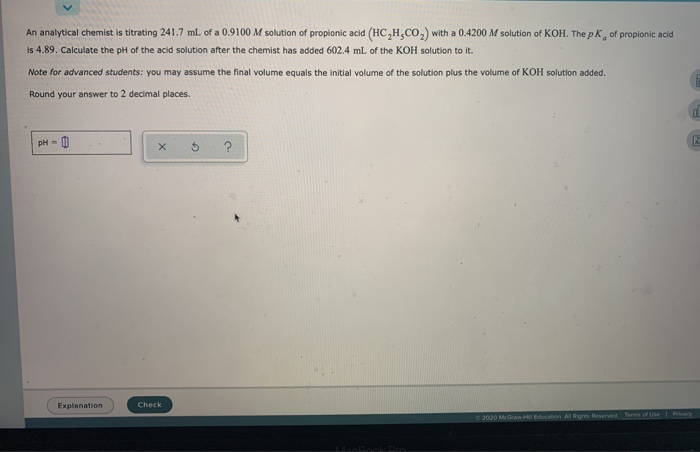calculating the ph of a weak acid titrated with a strong base An analytical chemist is titrating 241.7 ml of a 0.9100 M solution of propionic acid (HCH.CO) with a 0.4200 M solution of KOH. The pk of propionic acid is 4.89. Calculate the pH of the acid solution after the chemist has added 602.4 ml of the KOH solution to it. Note for advanced students: you may assume the final volume equals the initial volume of the solution plus...

• ### O ACIDS AND BASES Calculating the pH of a weak acid Utrated with a strong base...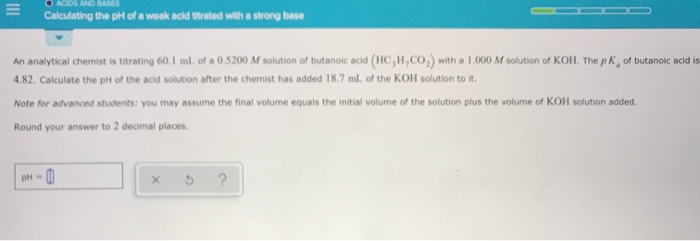O ACIDS AND BASES Calculating the pH of a weak acid Utrated with a strong base An analytical Chemist is titrating 60.1 ml of a 0.5200 M solution of butanolc acid (HC,H,CO) with a 1.000 M solution of KOH. The pk of butanoic acid is 4.82. Calculate the pH of the acid solution after the chemist has added 18.7 ml of the KOH solution to it. Note for advanced students you may assume the final volume equals the initial volume...

• ### ACIDS AND BASES Calculating the pH of a weak base titrated with a strong An analytical...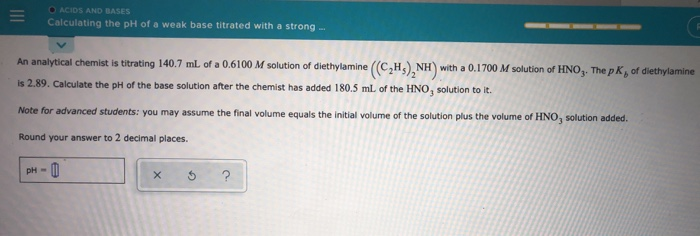ACIDS AND BASES Calculating the pH of a weak base titrated with a strong An analytical Chemist is titrating 140.7 mL of a 0.6100 M solution of diethylamine (CH), NH with a 0.1700 M solution of HNO. The pk, of diethylamine is 2.89. Calculate the pH of the base solution after the chemist has added 180.5 ml of the HNO, solution to it. Note for advanced students: you may assume the final volume equals the initial volume of the solution...

• ### An analytical chemist is titrating 188.5 mL of a 0.9900 M solution of propionic acid (HC,HCO,with...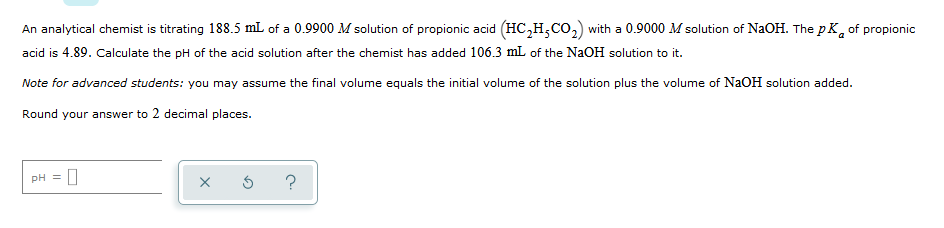An analytical chemist is titrating 188.5 mL of a 0.9900 M solution of propionic acid (HC,HCO,with a 0.9000 M solution of NaOH. The pK of propionic acid is 4.89. Calculate the pH of the acid solution after the chemist has added 106.3 mL of the NaOH solution to it. Note for advanced students: you may assume the final volume equals the initial volume of the solution plus the volume of NaOH solution added Round your answer to 2 decimal places....

• ### calculating the ph of a weak acid titrated with a strong base An analytical chemist is...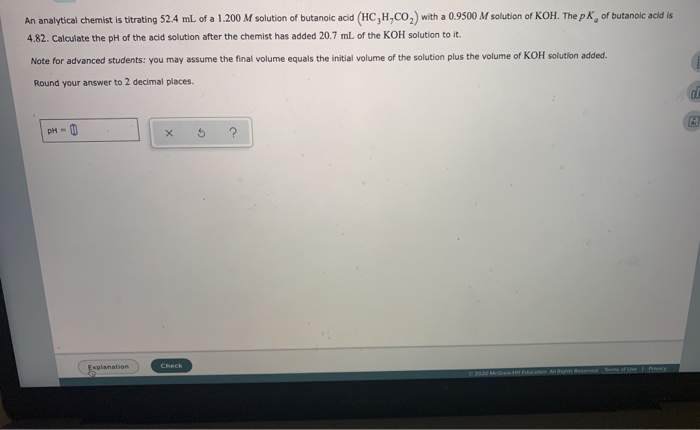calculating the ph of a weak acid titrated with a strong base An analytical chemist is titrating 52.4 ml of a 1.200 M solution of butanoic acid (HC,H,CO) with a 0.9500 M solution of KOH. The pK, of butanoic acid is 4.82. Calculate the pH of the acid solution after the chemist has added 20.7 mL of the KOH solution to it. Note for advanced students: you may assume the final volume equals the initial volume of the solution plus...

• ### - Calculating the pH of a weak acid titrated with a strong base An analytical chemist is titrating 123.0 ml. of a 0...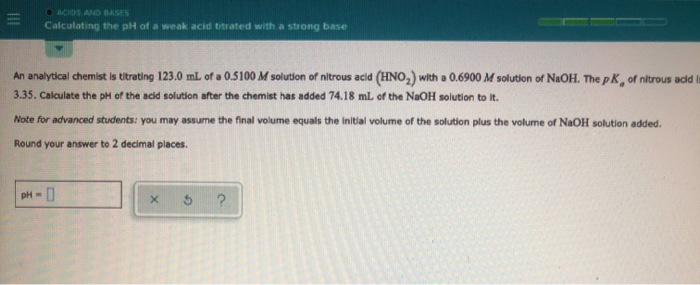- Calculating the pH of a weak acid titrated with a strong base An analytical chemist is titrating 123.0 ml. of a 0.5100 M solution of nitrous acid (HNO,) with a 0.6900 M solution of NaOH. The pk of nitrous add 3.35. Calculate the pH of the acid solution after the chemist has added 74.18 ml of the NaOH solution to it. Note for advanced students you may assume the final volume equals the initial volume of the solution plus...

• ### = O ACIDS AND BASES Calculating the pH at equivalence of a titration A chemist titrates...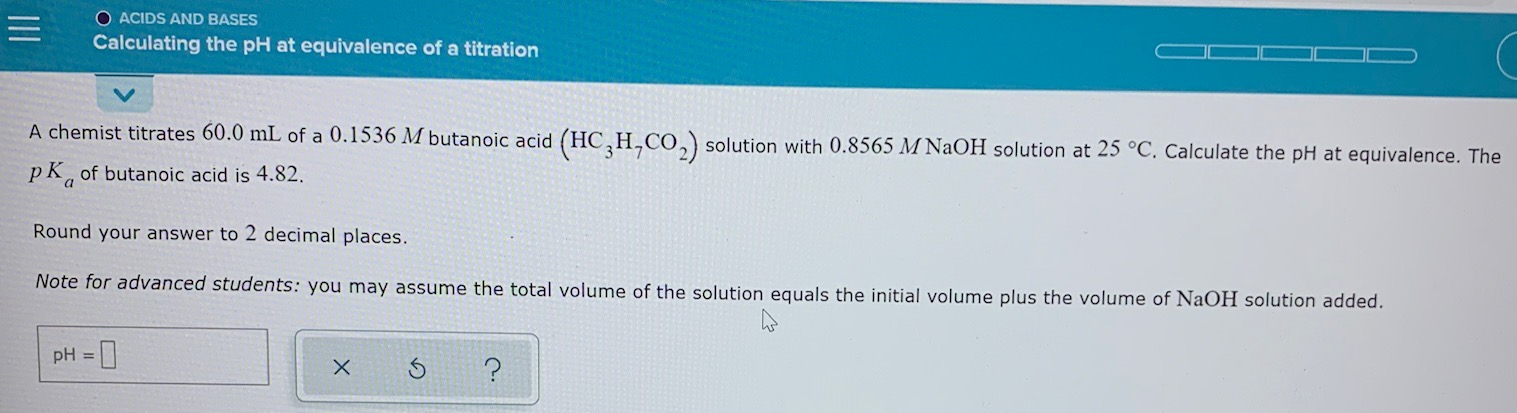= O ACIDS AND BASES Calculating the pH at equivalence of a titration A chemist titrates 60.0 mL of a 0.1536 M butanoic acid (HC,H,CO2) solution with 0.8565 M NaOH solution at 25 °C. Calculate the pH at equivalence. The pk, of butanoic acid is 4.82. Round your answer to 2 decimal places. Note for advanced students: you may assume the total volume of the solution equals the initial volume plus the volume of NaOH solution added. pH = x...

• ### = ACDS AND BASES Calculating the ph of a weak base titrated with a strong An...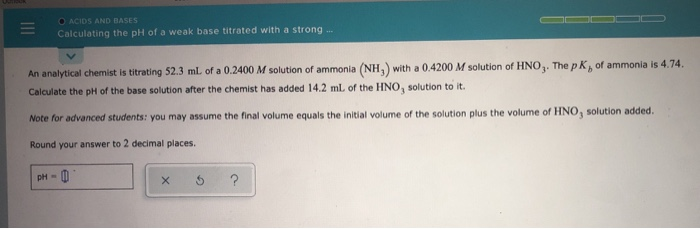= ACDS AND BASES Calculating the ph of a weak base titrated with a strong An analytical chemist is titrating 52.3 ml. of a 0.2400 M solution of ammonia (NH) with a 0.4200 M solution of HNO3. The pKof ammonia is 4.74. Calculate the pH of the base solution after the chemist has added 14.2 mL of the HINO, solution to it. Note for advanced students: you may assume the final volume equals the initial volume of the solution plus...